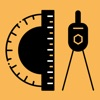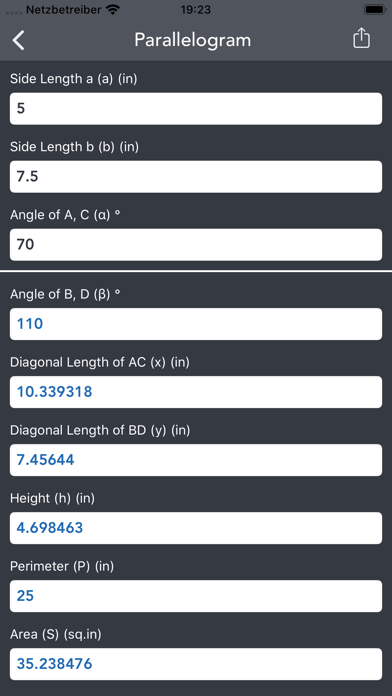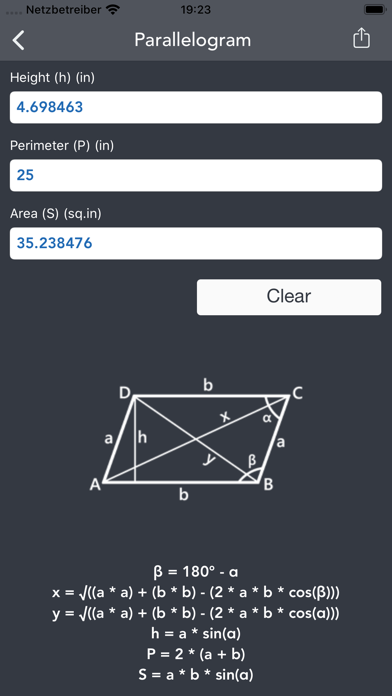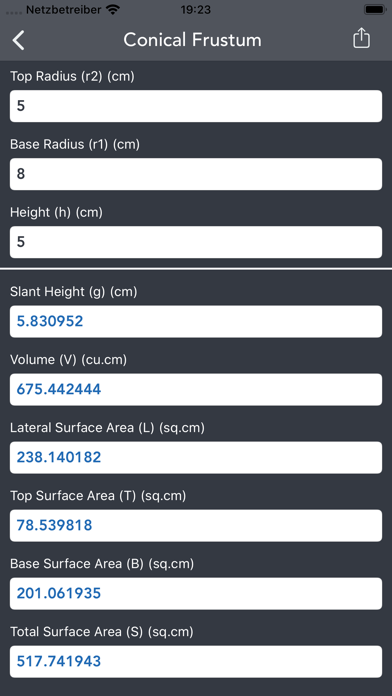## Geometry Calculator. - Lumos Educational App Store3.666671
Price -\$2.99
\$2.99

#### DESCRIPTION:

Ever wondered how many Geometrical shapes, planes and solids are there and how to calculate the parameters. Then this is the App for you. Geometry Calculator contains 26 shapes, planes and solids and 783 calculations for • Circle • Annulus • Ellipse • Torus • Square • Rectangle • Triangle • Triangle Theorems • Equilateral Triangle • Isosceles Triangle • Right Triangle • Parallelogram • Rhombus • Trapezoid • Polygon • Distance • 2D Distance • 3D Distance • Cube • Cuboid • Capsule • Cone • Cylinder • Conical Frustum • Sq

#### OVERVIEW:

Geometry Calculator. is a free educational mobile app By V PUGAZHENTHI.It helps students practice the following standards .

This page not only allows students and teachers download Geometry Calculator. but also find engaging Sample Questions, Videos, Pins, Worksheets, Books related to the following topics.

Developer: V PUGAZHENTHI

Developer URL: http://pugazh.net

Software Version: 2.3

Category: Education

Release Date: 2019-11-29T08:00:00Z#### Are you the Developer?EdSearch WebSearch